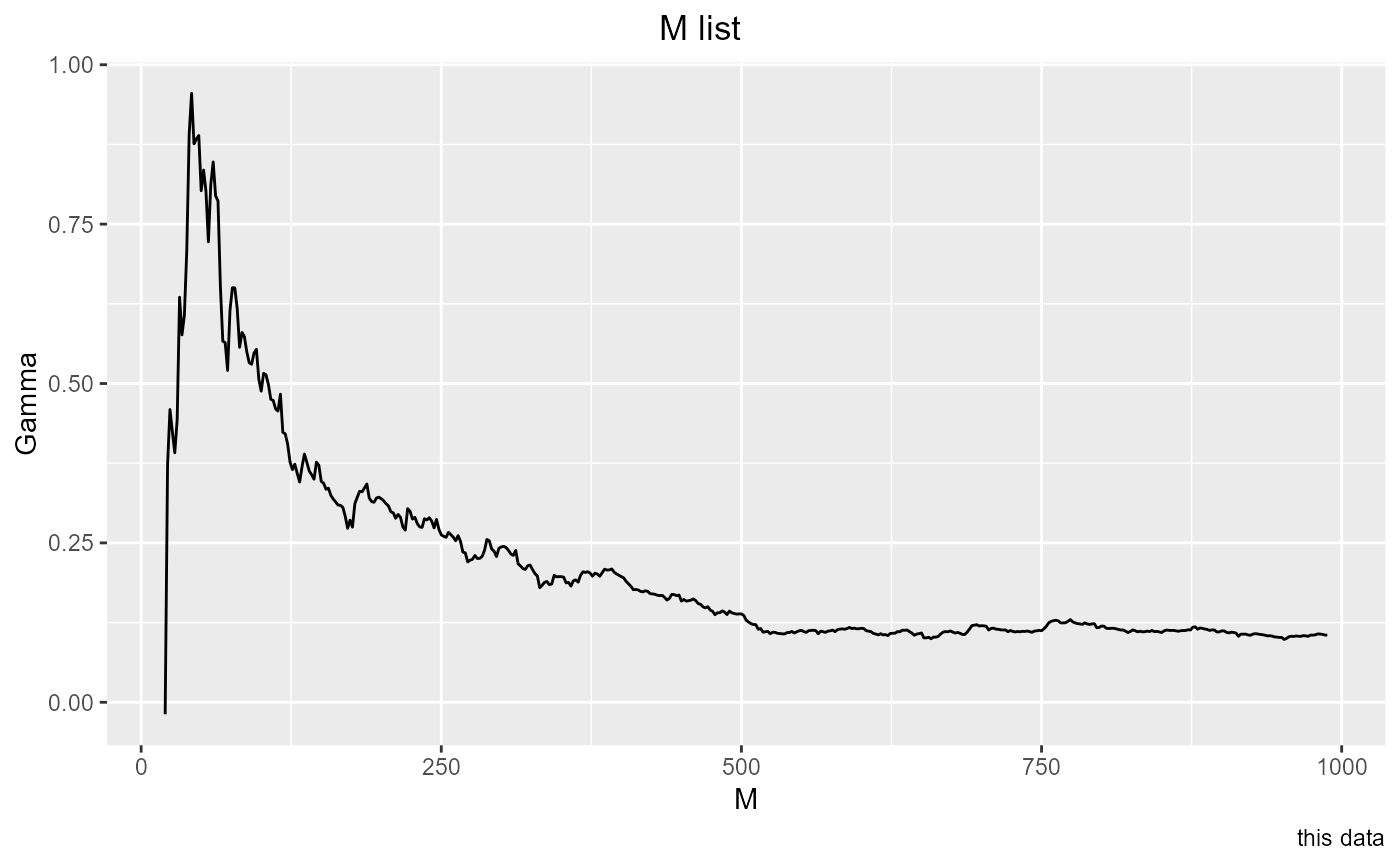Investigates the effect of sample size by calculating Gamma on larger and larger samples. Gamma will converge on the true noise in the relationship as sampling density on the function increases. get_Mlist produces a showing M values (sample sizes), and the associated Gammas and vratios. It produces a graph by default, and also returns an invisible data.frame. The successive samples are taken starting at the beginning of the inputs. There is no option to sort the input data; if you want the data to be randomized, do that before calling get_Mlist. The graph will become stable when the sample size is large enough. If the M list does not become stable, there is not enough data for either the Gamma test or a successful smooth model.

## Usage

get_Mlist(
predictors,
target,
plot = TRUE,
caption = "",
show = "Gamma",
from = 20,
to = length(target),
by = 20
)

## Arguments

predictors

A Numeric vector or matrix whose columns are proposed inputs to a predictive relationship

target

A Numeric vector, the output variable that is to be predicted

plot

A logical, set this to FALSE if you don't want the plot

caption

Character string to be used as caption for the plot

show

Character string, if it equals "vratio", vratios will be plotted, otherwise Gamma is plotted

from

Integer length of the first data sample, as passed to seq

to

Integer maximum length of sample to test, passed to seq

by

Integer increment in lengths of successive windows, passed to seq

## Value

An invisible data frame with three columns: M (a sample size), Gamma and the associated vratio. This is ordered by increasing M.

## Examples

he <- embed(henon_x, 13)
t <- he[ , 1]
p <- he[ ,2:13]
get_Mlist(p, t, by = 2, caption = "this data")